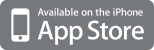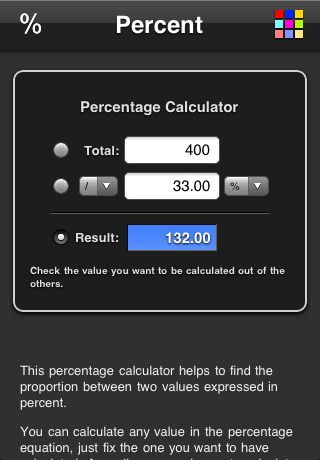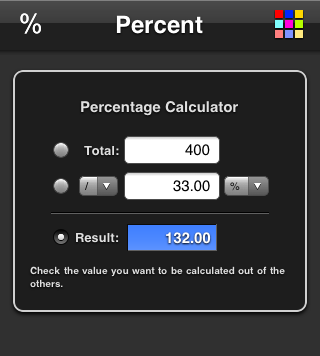## Sunday, November 15, 2009

### New in the App Store: Speed LimitCheck your speed and get warned if you drive faster than the speed limit to avoid speed tickets. Supports metric km/h and imperial mph speeds. There is an optional warning sound if your are speeding (with an adjustable tolerance).## Tuesday, November 3, 2009

### New in the App Store: PercentageThis percentage calculator helps to find the proportion between two values expressed in percent.

You can calculate any value in the percentage equation, just fix the one you want to have calculated. As well you can choose to calculate to percent increase or decrease between two values.### New Project: PercentageThis percentage calculator helps to find the proportion between two values expressed in percent.

You can calculate any value in the percentage equation, just fix the one you want to have calculated. As well you can choose to calculate to percent increase or decrease between two values.percent.speedymarks.com